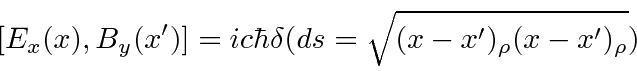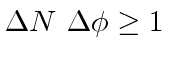## Uncertainty Relations and RMS Field Fluctuations

Since the fields are a sum of creation and annihilation operators, they do not commute with the occupation number operatorsObservables corresponding to operators which do not commute have an uncertainty principle between them. So we can't fix the number of photons and know the fields exactly. Fluctuations in the field take place even in the vacuum state, where we know there are no photons.

Of course the average value of the Electric or Magnetic field vector is zero by symmetry. To get an idea about the size of field fluctuations, we should look at the mean square value of the field, for example in the vacuum state. We compute.(Notice that we are basically taking the absolute square ofand that the orthogonality of the states collapses the result down to a single sum.)

The calculation is illustrative even though the answer is infinite. Basically, a term proportional tofirst creates one photon then absorbs it giving a nonzero contribution for every oscillator mode. The terms sum to infinity but really its the infinitesimally short wavelengths that cause this. Again, some cut off in the maximum energy would make sense.

The effect of these field fluctuations on particles is mitigated by quantum mechanics. In reality, any quantum particle will be spread out over a finite volume and its the average field over the volume that might cause the particle to experience a force. So we could average the Electric field over a volume, then take the mean square of the average. If we average over a cubic volume, then we find thatThus if we can probe short distances, the effective size of the fluctuations increases.

Even the E and B fields do not commute. It can be shown thatThere is a nonzero commutator of the two spacetime points are connected by a light-like vector. Another way to say this is that the commutator is non-zero if the coordinates are simultaneous. This is a reasonable result considering causality.

To make a narrow beam of light, one must adjust the phases of various components of the beam carefully. Another version of the uncertainty relation is that, whereis the phase of a Fourier component andis the number of photons.

Of course the Electromagnetic waves of classical physics usually have very large numbers of photons and the quantum effects are not apparent. A good condition to identify the boundary between classical and quantum behavior is that for the classical E&M to be correct the number of photons per cubic wavelength should be much greater than 1.

Jim Branson 2013-04-22